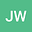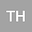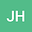MEASURING THE SEVERITY OF CLOSE ENCOUNTERS BETWEEN RINGED SMALL BODIES AND PLANETS
•••• +1
• Jeremy Wood,
• Tobias C Hinse,
• Jonti Horner,
• Awaiting Activation
Jeremy Wood

Corresponding Author:jeremy.wood@kctcs.edu

Author ProfileTobias C Hinse
Author ProfileJonti Horner
Author ProfileAwaiting Activation
University College of Southern Queensland
Author Profile## Abstract

????\revised????\accepted????\shorttitleRingsandCloseEncounters\shortauthorsWoodetal. The field of ringed Centaurs is only a few years old. Since Centaurs are known to regularly encounter the giant planets, it is of interest to explore the effect of a close encounter between a ringed Centaur and a giant planet on the ring structure. The severity of such an encounter depends on quantities such as the small body mass; velocity at infinity, $$v_{inf}$$; ring orbital radius, $$r$$; and encounter distance. In this work, we derive a formula for a critical distance at which the radial force is zero on a collinear ring particle in the four-body, circular restricted, planar problem. Numerical simulations of close encounters with Jupiter or Uranus in the three-body planar problem are made to experimentally determine the largest encounter distance, $$R$$, at which the effect on the ring is ”noticeable” using different values of small body mass, $$v_{inf}$$, and $$r$$. $$R$$ values are compared to the critical distance. We find that $$R$$ lies inside the critical distance for Centaurs with masses $$\ll$$ the mass of Pluto but can lie beyond it for Centaurs with the mass of Pluto and ring structure analogous to Chariklo’s. Changing the mass by a factor of almost 4 changed $$R$$ by $$\leq 0.2$$ tidal disruption distance, $$R_{td}$$. Effects on $$R$$ due to changes in $$v_{inf}$$, or $$r$$ are found to be $$\leq$$ 1.5$$R_{td}$$. $$R$$ values found using a four-body problem suggest that the critical distance might be useful as a first approximation of the constraint on $$R$$.